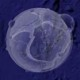## Scandinavia

NORSAR, as operator of the Norwegian National Data Centre (NDC) for the Comprehensive Test-Ban-Treaty (CTBT), publishes three main types of seismic bulletins. A continously updated (delayed about one hour behind real-time) automatic list of seismic events in northern Europe based on the GBF method, a monthly regional reviewed bulletin covering Fennoscandia and surrounding areas, and a monthly reviewed bulletin of teleseismic earthquakes and other seismic events.
These maps represent reviewed data.

## summary

mag1= 13 , mag2= 29 , mag3= 7
mag4= 0 , mag5= 0 total= 49

## summary

mag1= 10 , mag2= 50 , mag3= 3
mag4= 1 , mag5= 0 total= 64

## summary

mag1= 7 , mag2= 30 , mag3= 4
mag4= 8 , mag5= 0 total= 49

## summary

mag1= 5 , mag2= 33 , mag3= 6
mag4= 0 , mag5= 0 total= 44

## summary

mag1= 9 , mag2= 30 , mag3= 6
mag4= 2 , mag5= 0 total= 47

## summary

mag1= 10 , mag2= 33 , mag3= 5
mag4= 2 , mag5= 1 total= 51

## summary

mag1= 8 , mag2= 46 , mag3= 12
mag4= 0 , mag5= 0 total= 66

## summary

mag1= 5 , mag2= 35 , mag3= 4
mag4= 1 , mag5= 0 total= 45

## summary

mag1= 8 , mag2= 22 , mag3= 8
mag4= 0 , mag5= 0 total= 38

1.2.